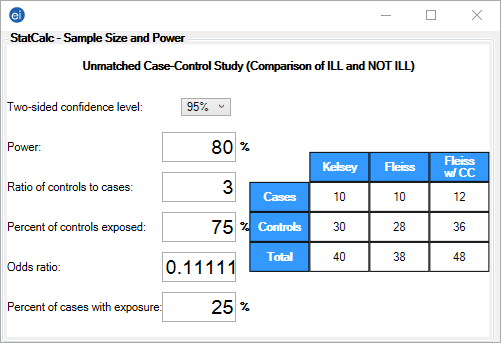# StatCalc: Statistical Calculators

StatCalc

### Unmatched Case-Control

The Unmatched Case-Control study calculates the sample size recommended for a study given a set of parameters and the desired confidence level.

#### Example

The following example demonstrates how to calculate a sample size for an unmatched case- control study. The application will show three different sample size estimates according to three different statistical calculations.

1. From the Epi Info™ main page, select StatCalc.
2. Select Unmatched Case-Control. The Unmatched Case-Control window opens.
3. Select the Two-sided confidence level of 95% from the drop-down list.
4. Enter the desired Power (80%) to detect a group difference at that confidence level.
5. Enter the ratio of controls to cases as 3. This is a single value and the proportion cannot be entered in the format, # of Unexposed : # of Exposed.
6. Enter the percentage outcome in the unexposed group 75%. This percentage represents the number of ill patients in the unexposed group.
7. Enter the percentage outcome in the exposed group 25%. This percentage represents the number of ill patients in the exposed group.
8. The Odds ratio automatically populates based on the values entered.
9. The output table shows three different estimates of sample size needed.Figure 10.12 Unmatched Case-Controls Study

Page last reviewed: September 16, 2022, 12:00 pm